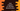# Write a Java program to calculate Discount Price## Java program to calculate the discount of a product in 3 ways:

In this tutorial, we will learn how to calculate the price of a product after the discount in `Java`. I will show you how to calculate the discount and the price after the discount in different ways.

### How to calculate the price after a discount:

To calculate the final price after the discount, we will have to multiply the actual price with the discount and subtract this from the original price e.g. if the actual price is 300 and the discount is 10%, the final price will be: `300 - (300 * 10/100)`. 10% discount will get you a discount of 10 for a product priced at 100. For a product priced at 1, you will get a discount of (10/100). And, for a product priced at 300, you will get a discount of 300*(10/100). We need to subtract the discount from the original price to get the final price.

If we consider it for `x%` discount,

• If the product price is `100` = The discount will be `x`
• If the product price is `1` = The discount will be `x/100`
• If the product price `y` = The discount will be `y * (x/100)`
• The final price of the product will be `y - (y * (x/100))`

We can use this algorithm to write a program to calculate the final price after a discount:

### Method 1: Java Program to calculate Discount with user input values:

``````import java.util.Scanner;

public class Example1 {

public static void main(String[] args) {
int price;
int discount;

try (Scanner sc = new Scanner(System.in)) {
System.out.println("Enter price of the product:");
price = sc.nextInt();

System.out.println("Enter the discount(%) of the product:");
discount = sc.nextInt();
}

int discountPrice = (price * discount) / 100;
int finalPrice = price - discountPrice;

System.out.println("Discount price is: " + discountPrice);
System.out.println("Final price is: " + finalPrice);
}
}``````

• The integer variables `price` and `discount` are used to assign the price and the discount percentage of the product.
• It uses the `Scanner` class to read the inputs from the user. The `nextInt()` method is used to read the price and discount values entered by the user. It assigns the values to the `price` and `discount` variables.
• The discounted price is calculated with the formula we discussed above and it is assigned to the `discountPrice` variable. The final price is calculated by subtracting the `discountPrice` value from the original `price` of the product. It is assigned to the `finalPrice` variable.
• The last two lines are printing the calculated discount price and the final price.

#### Sample Output:

If you run the above program, it will give outputs as below:

``````Enter price of the product :
400
Enter the discount(%) of the product :
10
Discount price is: 40
Final price is: 360``````

### Method 2: How to use a different method to calculate the discounted price and the final price:

Another way to solve this example is by using two separate methods. The `main` method will call these methods to calculate the discounted price and the final price.

``````import java.util.Scanner;

public class Example2 {

static int getDiscount(int price, int discount) {
return (price * discount) / 100;
}

static int getFinalPrice(int price, int discountPrice) {
return price - discountPrice;
}

public static void main(String[] args) {
int price;
int discount;

try (Scanner sc = new Scanner(System.in)) {
System.out.println("Enter price of the product :");
price = sc.nextInt();

System.out.println("Enter the discount(%) of the product :");
discount = sc.nextInt();
}

int discountPrice = getDiscount(price, discount);
int finalPrice = getFinalPrice(price, discountPrice);

System.out.println("Discount price is: " + discountPrice);
System.out.println("Final price is: " + finalPrice);
}
}``````

• We have created two new methods `getDiscount` and `getFinalPrice` to calculate the discount and the final price.
• The `main` method is calling these methods to calculate the values.
• These are `static` methods. You can also move these methods to a different class to access these from different parts of your program.

It will print similar results.

``````Enter price of the product :
200
Enter the discount(%) of the product :
20
Discount price is: 40
Final price is: 160``````

### Method 3: How to use a different class to hold the values of the discount and final price:

In this method, we will use a separate model class to hold the discount and final price of the product. We can avoid writing two methods to calculate the discount and the final price with this approach.

``````import java.util.Scanner;

class DiscountModel {
int finalPrice;
int discountPrice;

public DiscountModel(int finalPrice, int discountPrice) {
this.finalPrice = finalPrice;
this.discountPrice = discountPrice;
}
}

public class Example3 {

static DiscountModel getDiscount(int price, int discount) {
int discountPrice = (price * discount) / 100;
int finalPrice = price - discountPrice;

return new DiscountModel(finalPrice, discountPrice);
}

public static void main(String[] args) {
int price;
int discount;

try (Scanner sc = new Scanner(System.in)) {
System.out.println("Enter price of the product :");
price = sc.nextInt();

System.out.println("Enter the discount(%) of the product :");
discount = sc.nextInt();
}

DiscountModel result = getDiscount(price, discount);

System.out.println("Discount price is: " + result.discountPrice);
System.out.println("Final price is: " + result.finalPrice);
}
}``````

• The `DiscountModel` class is used as the model class. The `getDiscount` method returns an object of `DiscountModel`. It calculates the discount and the final price of the product and assigns these to the `discountPrice` and `finalPrice` variables of the `DiscountModel` object.
• The `main` method is assigning the calculated value to the `result` variable and it prints the values of the member variables of the `DiscountModel` class.